#Rational Zeros of PolynomialsThis section deals with polynomials which have integer coefficients only.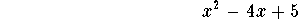is a polynomial with integer coefficients, the polynomial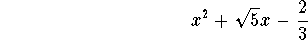does not have only integer coefficients!

You will learn how to find all those roots of such polynomials, which are rational numbers, such as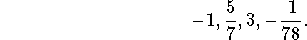This is not merely an esoteric exercise. Suppose, you would like to factor the polynomial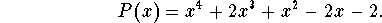There is a general formula for the roots of a polynomial of degree 4, but it is VERY tedious to apply. It so happens that in this case x=1 and x=-1 are two rational zeros (=two roots, which are rational numbers). Do you remember how to check this? To say that x=1 is a root of P(x) is the same as saying that P(1)=0.

This means that (x-1)(x+1) divides evenly into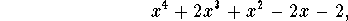so after a polynomial long division, we are left with factoring a quadratic polynomial, which in this case turns out to have the complex roots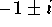. (Check the details!)

#### An introductory example.

How did I know that x=1 and x=-1 were roots of the polynomial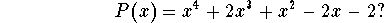Suppose the polynomial has a rational root, let's call it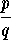. I will assume that p and q are coprime, i.e., the fraction is reduced to lowest terms.

What we will be doing is somewhat similar to "factoring by guessing" of quadratic polynomials.

Since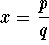is assumed to be a root of P(x), we know that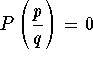: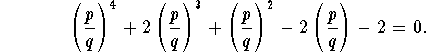If we multiply both sides by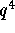, we obtain: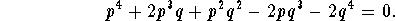Transfer the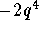to the other side, and factor out a p on the left: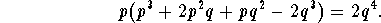Now the left side is divisible by p; consequently,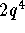is divisible by p. Since p does not divide q, it does not divide, so p divides 2, i.e.,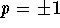or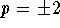.

Start again:This time we transfer all but the first term to the other side, and factor out a q on the right side: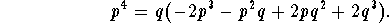Now the right side is divisible by q; consequently, the left side, the term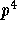is divisible by q. Since p and q are coprime, this means that q divides 1, i.e.,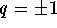.

What have we shown? Every rational rootof P(x) is one of the following 4 choices: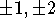. (these are the only four numbers with p dividing 2 and q dividing 1).

We can now check each one of them: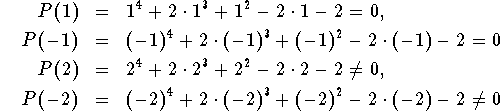Consequently, the polynomial has 2 rational roots: x=1 and x=-1 are the only rational zeros of the polynomial P(x).

#### The Rational Zero Test.

Note that in our example the leading coefficient was 1, and the constant term was -2. These were really the only two pieces of information we needed to find all rational zeros:

 Suppose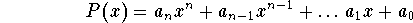is a polynomial with integer coefficients, andis a rational zero of P(x). Then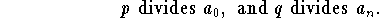#### Another example.

Find all rational zeros of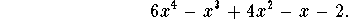The leading coefficient is 6, the constant coefficient is -2. If this polynomial has rational zeros, then p divides -2 and q divides 6. Thus we have the following choices for p: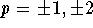; for q our choices are: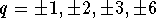.

The candidates for rational zeros are (in decreasing order of magnitude):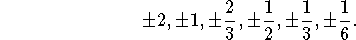Now you have to check which (if any) of these 12 values are actually roots of P(x). Doing this by hand will be tedious. Having a graphing calculator comes in handy; here is the graph of P(x) for the region we are interested in, for x-values between -2 and 2.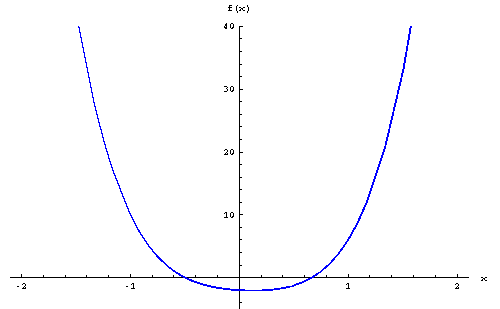There are only roots close to x=-1/2 and close to x=2/3. This reduces our list of candidates to just two; plugging these values into the polynomial, we see that P(-1/2)=0 and P(2/3)=0, so both are indeed rational zeros. (You have to perform this last step! It could be that P(x) does not have a rational root at 2/3, but an irrational root very close to 2/3. You would not be able to distinguish between these two cases just by looking at the graph.)

#### Example 3.

Factor the polynomial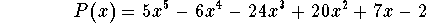completely over the real numbers.

Let's find all rational zeros: they all have the form, where p divides the constant term -2, and q divides the leading coefficient 5. The choices for p are, the choices for q are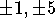. This leaves eight possible choices for rational zeros: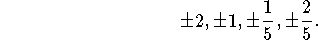If we plug these values into the polynomial P(x), we obtain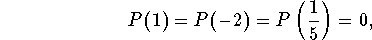while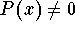for the other five choices.

Consequently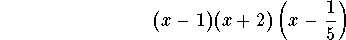divides evenly into P(x). If we perform polynomial long division, we see that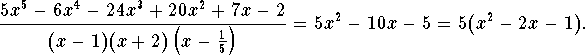Using the quadratic formula we find, that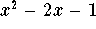has the real roots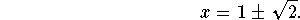Putting it all together, we obtain the following factorization for P(x):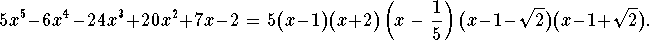#### Exercise 1.

Find all rational zeros of the polynomial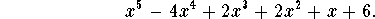#### Exercise 2.

Find all rational zeros of the polynomial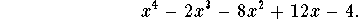#### Exercise 3.

Find all rational zeros of the polynomial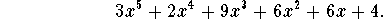#### Exercise 4.

Factor the polynomial in Exercise 3 completely (a) over the real numbers, (b) over the complex numbers.

#### Exercise 5.

Show the following: If a polynomial has integer coefficients and its leading coefficient is 1, then all of its rational zeros are in fact integers.[Back] [Next]
[Algebra] [Trigonometry] [Complex Variables]
[Calculus] [Differential Equations] [Matrix Algebra]S.O.S MATHematics home page

Do you need more help? Please post your question on our S.O.S. Mathematics CyberBoard.Helmut Knaust